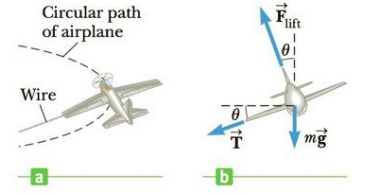Chapter 7, Problem 60AP

Chapter
Section
Textbook Problem

A model airplane of mass 0.750 kg flies with a speed of 35.0 m/s in a horizontal circle at the end of a 60.0-m control wire as shown in Figure P7.60a. The forces exerted on the airplane are shown in Figure P7.60b; the tension in the control wire, θ = 20.0° inward from the vertical. Compute the tension in the wire, assuming the wire makes a constant angle of θ = 20.0° with the horizontal.Figure P7.60

To determine
The tension in the wire.

Explanation

Given info: The mass of the model airplane is 0.750kg . The speed of the airplane in the horizontal circle is 35.0ms-1 . The length of the control wire is 60.0m . The wire makes a constant angle of 20.0° with the horizontal.

Explanation:

As the plane is moving in the horizontal circle, there is no acceleration in the vertical direction. The net force in the vertical direction will be zero.

FliftcosθTsinθmg=0

• Flift is the lift force
• θ is the angle between the horizontal and the plane
• T is the tension force
• m is the mass of the airplane
• g is the acceleration due to gravity

Re-arranging to find an expression for F ,

F=Ttanθ+mgcosθ       (1)

The centripetal acceleration for the plane is provided by horizontal component of the tension and the horizontal component of the lift force,

Fsinθ+Tcosθ=mv2r       (2)

Combining (1) and (2),

(Ttanθ+mgcosθ)sinθ+Tcosθ=mv2r

From the above expression the expression for the tension force will be,

T=m(v2r)mgtanθsinθ

Still sussing out bartleby?

Check out a sample textbook solution.

See a sample solution

The Solution to Your Study Problems

Bartleby provides explanations to thousands of textbook problems written by our experts, many with advanced degrees!

Get Started